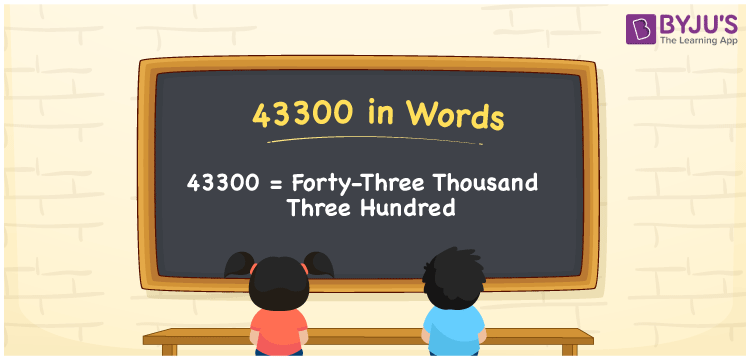# 43300 in Words

43300 in words can be written as Forty-Three Thousand Three Hundred. The name of 43300 can be written using the place value chart. If you pay the yearly fees of Rs. 43300 to the college, then you can say that “I paid yearly fees of Forty-Three Thousand Three Hundred Rupees to the college”. Numbers in words are explained in a simple language to help students learn the concepts well in their higher education levels. 43300 can be read as “Forty-Three Thousand Three Hundred” in English.

 43300 in words Forty-Three Thousand Three Hundred Forty-Three Thousand Three Hundred in Numbers 43300

## 43300 in English Words## How to Write 43300 in Words?

Five digits are present in the number 43300 which is shown in the form of a place value chart below.

 Ten Thousands Thousands Hundreds Tens Ones 4 3 3 0 0

43300 in expanded form can be written as:

4 x Ten Thousand + 3 x Thousand + 3 × Hundred + 0 × Ten + 0 × One

= 4 x 10000 + 3 x 1000 + 3 x 100 + 0 x 10 + 0 x 1

= 40000 + 3000 + 300

= 43300

= Forty-Three Thousand Three Hundred

Hence, 43300 in words is written as Forty-Three Thousand Three Hundred.

43300 is a natural number that precedes 43301 and succeeds 43299.

43300 in words – Forty-Three Thousand Three Hundred

Is 43300 an odd number? – No

Is 43300 an even number? – Yes

Is 43300 a perfect square number? – No

Is 43300 a perfect cube number? – No

Is 43300 a prime number? – No

Is 43300 a composite number? – Yes

## Frequently Asked Questions on 43300 in Words

### How to write 43300 in words?

43300 can be written in words as “Forty-Three Thousand Three Hundred”.

### Write Forty-Three Thousand Three Hundred in numbers.

Forty-Three Thousand Three Hundred in numbers can be written as 43300.

### Is 43300 an even number?

43300 is an even number as it is completely divisible by 2.